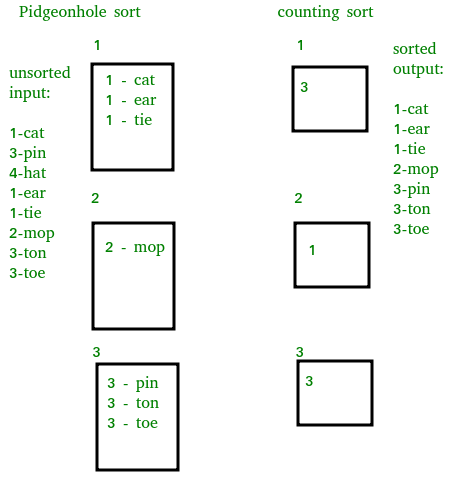Open In App
Related Articles

# Pigeonhole Sort

Pigeonhole sorting is a sorting algorithm that is suitable for sorting lists of elements where the number of elements and the number of possible key values are approximately the same.
It requires O(n + Range) time where n is number of elements in input array and ‘Range’ is number of possible values in array.
Working of Algorithm :

1. Find minimum and maximum values in array. Let the minimum and maximum values be ‘min’ and ‘max’ respectively. Also find range as ‘max-min+1’.

2. Set up an array of initially empty “pigeonholes” the same size as of the range.
3. Visit each element of the array and then put each element in its pigeonhole. An element arr[i] is put in hole at index arr[i] – min.
4. Start the loop all over the pigeonhole array in order and put the elements from non- empty holes back into the original array.

Comparison with Counting Sort :
It is similar to counting sort, but differs in that it “moves items twice: once to the bucket array and again to the final destination “.## C++

 `/* C++ program to implement Pigeonhole Sort */``#include ``using` `namespace` `std;` `/* Sorts the array using pigeonhole algorithm */``void` `pigeonholeSort(``int` `arr[], ``int` `n)``{``    ``// Find minimum and maximum values in arr[]``    ``int` `min = arr, max = arr;``    ``for` `(``int` `i = 1; i < n; i++)``    ``{``        ``if` `(arr[i] < min)``            ``min = arr[i];``        ``if` `(arr[i] > max)``            ``max = arr[i];``    ``}``    ``int` `range = max - min + 1; ``// Find range` `    ``// Create an array of vectors. Size of array``    ``// range. Each vector represents a hole that``    ``// is going to contain matching elements.``    ``vector<``int``> holes[range];` `    ``// Traverse through input array and put every``    ``// element in its respective hole``    ``for` `(``int` `i = 0; i < n; i++)``        ``holes[arr[i]-min].push_back(arr[i]);` `    ``// Traverse through all holes one by one. For``    ``// every hole, take its elements and put in``    ``// array.``    ``int` `index = 0;  ``// index in sorted array``    ``for` `(``int` `i = 0; i < range; i++)``    ``{``       ``vector<``int``>::iterator it;``       ``for` `(it = holes[i].begin(); it != holes[i].end(); ++it)``            ``arr[index++]  = *it;``    ``}``}` `// Driver program to test the above function``int` `main()``{``    ``int` `arr[] = {8, 3, 2, 7, 4, 6, 8};``    ``int` `n = ``sizeof``(arr)/``sizeof``(arr);` `    ``pigeonholeSort(arr, n);` `    ``printf``(``"Sorted order is : "``);``    ``for` `(``int` `i = 0; i < n; i++)``        ``printf``(``"%d "``, arr[i]);` `    ``return` `0;``}`

## Java

 `/* Java program to implement Pigeonhole Sort */` `import` `java.lang.*;``import` `java.util.*;` `public` `class` `GFG``{``    ``public` `static` `void` `pigeonhole_sort(``int` `arr[],``                                           ``int` `n)``    ``{``        ``int` `min = arr[``0``];``        ``int` `max = arr[``0``];``        ``int` `range, i, j, index;` `        ``for``(``int` `a=``0``; a max)``                ``max = arr[a];``            ``if``(arr[a] < min)``                ``min = arr[a];``        ``}` `        ``range = max - min + ``1``;``        ``int``[] phole = ``new` `int``[range];``        ``Arrays.fill(phole, ``0``);` `        ``for``(i = ``0``; i``0``)``                ``arr[index++]=j+min;` `    ``}` `    ``public` `static` `void` `main(String[] args)``    ``{``        ``GFG sort = ``new` `GFG();``        ``int``[] arr = {``8``, ``3``, ``2``, ``7``, ``4``, ``6``, ``8``};` `        ``System.out.print(``"Sorted order is : "``);` `        ``sort.pigeonhole_sort(arr,arr.length);``        ` `        ``for``(``int` `i=``0` `; i`

## Python3

 `# Python program to implement Pigeonhole Sort */` `# source code : "https://en.wikibooks.org/wiki/``#   Algorithm_Implementation/Sorting/Pigeonhole_sort"``def` `pigeonhole_sort(a):``    ``# size of range of values in the list``    ``# (ie, number of pigeonholes we need)``    ``my_min ``=` `min``(a)``    ``my_max ``=` `max``(a)``    ``size ``=` `my_max ``-` `my_min ``+` `1` `    ``# our list of pigeonholes``    ``holes ``=` `[``0``] ``*` `size` `    ``# Populate the pigeonholes.``    ``for` `x ``in` `a:``        ``assert` `type``(x) ``is` `int``, ``"integers only please"``        ``holes[x ``-` `my_min] ``+``=` `1` `    ``# Put the elements back into the array in order.``    ``i ``=` `0``    ``for` `count ``in` `range``(size):``        ``while` `holes[count] > ``0``:``            ``holes[count] ``-``=` `1``            ``a[i] ``=` `count ``+` `my_min``            ``i ``+``=` `1``            `  `a ``=` `[``8``, ``3``, ``2``, ``7``, ``4``, ``6``, ``8``]``print``(``"Sorted order is : "``, end ``=` `' '``)` `pigeonhole_sort(a)``        ` `for` `i ``in` `range``(``0``, ``len``(a)):``    ``print``(a[i], end ``=` `' '``)``   `

## C#

 `// C# program to implement``// Pigeonhole Sort``using` `System;` `class` `GFG``{``public` `static` `void` `pigeonhole_sort(``int` `[]arr,``                                   ``int` `n)``{``    ``int` `min = arr;``    ``int` `max = arr;``    ``int` `range, i, j, index;` `    ``for``(``int` `a = 0; a < n; a++)``    ``{``        ``if``(arr[a] > max)``            ``max = arr[a];``        ``if``(arr[a] < min)``            ``min = arr[a];``    ``}` `    ``range = max - min + 1;``    ``int``[] phole = ``new` `int``[range];``    ` `    ``for``(i = 0; i < n; i++)``    ``phole[i] = 0;` `    ``for``(i = 0; i < n; i++)``        ``phole[arr[i] - min]++;` `    ` `    ``index = 0;` `    ``for``(j = 0; j < range; j++)``        ``while``(phole[j] --> 0)``            ``arr[index++] = j + min;` `}` `// Driver Code``static` `void` `Main()``{``    ``int``[] arr = {8, 3, 2, 7,``                 ``4, 6, 8};` `    ``Console.Write(``"Sorted order is : "``);` `    ``pigeonhole_sort(arr,arr.Length);``    ` `    ``for``(``int` `i = 0 ; i < arr.Length ; i++)``        ``Console.Write(arr[i] + ``" "``);``}``}` `// This code is contributed``// by Sam007`

## Javascript

 ``

Output

`Sorted order is : 2 3 4 6 7 8 8 `

Pigeonhole sort has limited use as requirements are rarely met. For arrays where range is much larger than n, bucket sort is a generalization that is more efficient in space and time.

Complexity Analysis of  Pigeonhole Sort:

The time complexity of Pigeonhole Sort is O(n + range), where n is the number of elements in the array and range is the range of the input data (i.e., the difference between the maximum and minimum values in the array).

In the given implementation, the algorithm first finds the minimum and maximum values in the array, which takes O(n) time. Then, it calculates the range, which takes constant time. Next, it creates an array of vectors of size equal to the range, which takes constant time. Then, it traverses the input array and puts each element into its respective hole, which takes O(n) time. Finally, it traverses all the holes and puts their elements into the output array in order, which takes O(range) time.

Therefore, the overall time complexity of the algorithm is O(n + range). In the worst case, when the range is significantly larger than the number of elements in the array, the algorithm can be inefficient. However, it can be useful for sorting integer arrays with a relatively small range.

Auxiliary Space: O(range)

• It is a non-comparison based sort making it faster in application.
• It is a stable sorting algorithm.
• It performs sorting in linear time.